# Quiz 11: Heat

Physics & Astronomy

Calorie and calorie are the units of energy. One calorie defined as mount of heat needed to rise the temperature of 1 g of water where as one Calories is used when the amount of heat needed to rise the temperature of 1 kg of water. Therefore, the relation between calorie and Calorie is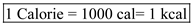.

State the rating of the window air conditioner in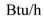.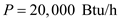Convert the unit of energy from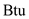to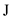.Convert the unit of time from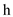to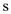.Now calculate the rating of the air conditioner.Therefore, rating of air conditioner is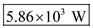.

The expression for the latent heat is,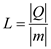Here,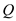is the heat energy and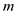is the mass of the substance. The heat energy needed to change the phase of the substance per kilogram of mass is called as latent heat. The SI unit of the mass is kilogram and the SI unit of heat energy is joule. The heat energy per unit mass is defined as the latent heat. So, the unit of the latent heat is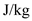. Hence, options (a), (b), and (c) are incorrect. Therefore, option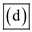is correct.